### PAPER:

The Barone-Adesi Whaley  formula to price American options revisited

.

1. Abstract

In   we present a method to solve the American  option pricing problem in the Black Scholes framework that generalizes the Barone-Adesi,  Whaley method . An auxiliary parameter is introduced in the American option pricing problem.   Power series expansions in this parameter of the option price and of the corresponding free boundary are derived. These series expansions have the Baroni-Adesi, Whaley formula as zero-th order term. The coefficients of the option price series are explicit formulae. The partial sums of the free boundary series  are determined numerically as solutions of  nonlinear   equations that depend from the time variable as a parameter.   Numerical experiments on test problems suggest that the series expansions derived are convergent. The evaluation on a grid of values of the independent variables of the truncated  series expansions  is easily parallelizable.  The cost of computing the n-th order approximations of the series expansions is approximately proportional to n as n goes to infinity. The results obtained on a set of test problems with the first and second order  approximations deduced from the series expansions of the option prices and of the corresponding  free boundaries  outperform in accuracy  and/or in computational cost the results obtained with  alternative methods (, , ). In particular  when we consider options with maturity time between three and ten years   and positive cost of carrying parameter (i.e. when the continuous dividend yield is smaller than the risk free interest rate)  the second order approximation of the free boundary obtained from the series expansions  improves substantially the free boundary obtained with the Barone-Adesi, Whaley formula .

This website contains material  including animations, an interactive application and an app that helps the understanding of  . A  general reference to the work of the authors and of their coauthors in mathematical finance is the website:

2. A perturbation expansion to solve the American option pricing problem

We present a method to solve the American option pricing problem that generalizes the Barone-Adesi, Whaley method . To fix the ideas we consider options on commodities as done in . Let t be a real variable that denotes time and St, t>0, be a real stochastic process that models the commodity price. Under the risk neutral measure we assume that St, t>0, satisfies the following stochastic differential equation:

 dSt=b St dt+s  St  dzt ,   t>0,

(1)

where b, s are real parameters, zt, t>0, is a standard Wiener process such that z0=0 and dzt is its stochastic differential. The parameter s>0 is the volatility or instantaneous standard deviation and the parameter b is the cost of carrying. In the most common situations we have b=r-d where r>0 is the risk free interest rate and d>0 is the continuous dividend yield. Equation (1) is called Black Scholes asset price dynamic equation.

We study the American call option pricing problem. The results obtained for the American call option pricing problem are easily extended to the American put option pricing problem.  Let us consider  an American call option with maturity time T>0 and strike price E>0 on a commodity whose price St, t>0, satisfies (1). We denote with t=T-t, 0 £ t £ T, the time to maturity, with S the commodity price. Let CA(S,t), 0<S<S* (t), 0 £ t £ T, and S* (t), 0<t<T, be respectively the price and the free boundary of the  option studied. The American call option pricing problem written in the independent variables S, t for the unknown functions CA, S* is given by: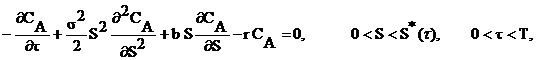(2)

with boundary conditions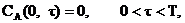(3)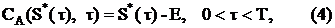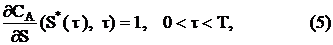and initial condition

 CA(S,0)=max(S-E,0),    0

Following  Barone-Adesi and Whaley  we look for a solution of problem  (2), (3) , (4),  (5), (6)  given by the sum of the price CE(S,t), S>0, 0<t<T, of the European call option having strike price E, maturity time T and parameters  of the Black Scholes asset price dynamic equation b and s and of a quantity e(S,t), 0<S<Sf(t), 0<t<T, called early exercise premium.  Moreover  we assume that the early exercise premium e  is  written as follows (see ):

 e(S,t)=K(t)f(S,K(t)),    0

where K(t)=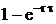, 0<t<T, and f is an auxiliary function to be determined. That is we assume that the American call option price is given by:

 CA(S,t)=CE(S,t)+e(S,t)=CE(S,t)+K(t)f(S,K(t)),

0<S<S*

(t),  0<t< T.                          (8)

Note that  the price CE is defined for S>0, 0<t<T,  has a closed form expression known as Black Scholes formula and  satisfies (2), (3), (6).  From  K(0)=0 and the fact that CE satisfies  (6) it follows that when f is well behaved in t=0 the option price CA given by (8) satisfies (6). Substituting  (8)  in  (2), (3), (4), (5) and using the fact that CE  satisfies (2), (3), (6) we obtain the following problem for the unknown functions f, S* :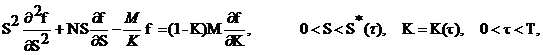(9)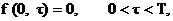(10)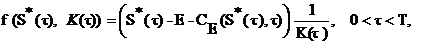(11)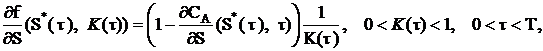(12)

where M=2r/s2 and N=2b/s2 .   Note that K=K(t), 0<t<T, and that in the formulation of problem (9), (10), (11), (12) we use both K and  t as variables.

In  Barone-Adesi and Whaley dropped the term (1-K)M( f/ K) in (9) and solved the remaining problem.  The idea of dropping the term (1-K)M( f/ K) in (9)   is ingenious and fruitful.  In fact the term (1-K)M( f/ K)  tends to zero when t goes to zero and when t goes to T and T goes to infinity, this implies that the Barone-Adesi, Whaley formula is accurate when we consider options with  short or with long  maturity time. The revisited Barone-Adesi, Whaley formula presented in  is based on the introduction of an auxiliary parameter in the right hand side of (9)  and in the use of  power series expansions in this parameter to approximate the price and  the  free boundary of the American option pricing problem considered. The zero-th order terms of these expansions are the Barone-Adesi, Whaley formula. Specifically  equation  (9) is replaced with the following equation: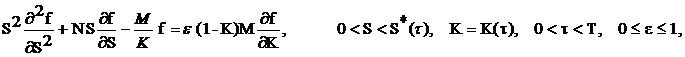(13)

where e is the announced auxiliary parameter.  We study problem (13), (10), (11), (12) and we consider the following series expansions in powers of e of the function f and of the corresponding free boundary S* :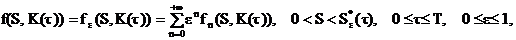(14)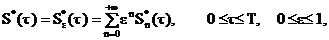(15)

where the functions  fn,  Sn* , n=0,1,¼, are independent of e. In  using  (13), (10), (11), (12) we determine the coefficients of the option price series expansion (14) and the partial sums of the free boundary series expansion (15). The coefficients of the option price series expansion are explicit formulae that contain the free boundary. The partial sums of the free boundary series expansion are determined numerically solving nonlinear equations that contain the time to maturity t as a parameter, 0≤t ≤T.

Let f be well behaved in t =0. In this case problem (13),  (10), (11), (12) when e=1 is equivalent to the American call option pricing problem  (2),  (3),  (4),  (5), (6), when e=0 is the problem solved by Barone-Adesi, Whaley in  and when e à 0+ is a singular perturbation problem. In fact note that when e=0 the higher order derivative with respect to K contained in  (13)  is multiplied by zero. This fact suggests that when eà 0+ an expansion of fe, Se* in powers of e with base point e=0 does not hold uniformly in K and S for 0<K< 1-e-rT , 0<S<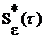,  0 £ t £ T, due to the presence of a boundary layer in K=0.  In singular perturbation theory this kind of problems is studied using the method of matched asymptotic expansions. That is when e à 0+ two series (i.e. inner and outer expansions) appropriately matched are necessary to get a uniform approximation of the solution of problem  (13) , (10), (11) , (12)  when 0<K<1-e-r t,  0<S<S* (t), 0<t<T.

However in finance the solution of problem (13), (10), (11), (12)  is relevant only when e=1. The behaviour of the solution of problem (13), (10), (11), (12)  when eà 0+  has no interest in finance.

We avoid the study of problem (13), (10), (11), (12) when eà 0+ . In fact in   we impose to the truncated series expansions (14), (15) the boundary conditions (11) , (12) in e=1 instead of imposing them in e=0 order by order in perturbation theory to the inner and outer expansions of the matched asymptotic expansion method (see ). Note that the series expansions (14) ,  (15)  are a kind of outer expansion of the solution of problem (13), (10), (11), (12). The functions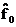,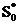, that is the zero-th order terms of the series expansions (14) ,  (15)  are the Barone-Adesi, Whaley solution of the American option pricing problem.

3. Some numerical experiments

Let us present some numerical results obtained on a set of test problems with the solution method sketched in Section 2 (see ).

We use the trinomial tree method  with nT = 1000 time steps to compute the ``true value" of the option prices considered in our experiments. The choice nT = 1000 guarantees four correct significant digits in the option prices computed in the experiments. For American call options the ``true value" of the free boundaries considered  is computed solving numerically the integral equation satisfied by the free boundary presented in ,  and recalled in .

We begin our numerical experiments studying some test problems taken from  Section C.4. These test problems consider options on long-term U.S. Treasury bonds (time to maturity up to three years) and long term care insurance inflation options (time to maturity up to ten years and beyond).

In the first experiment we use the Black Scholes parameter values of  Table V. That is we consider the following three sets of parameter values: r = 0.08, s = 0.2, and b = -0.04, or b = 0.0 or b = 0.04. For each set of Black Scholes parameter values we consider three call options with strike price E = 100 and maturity time T = 3, or T = 5, or T = 10. The time T is expressed in years.

Table 1 shows the free boundary approximations of the American call option pricing problems specified above when t = T = 3,5,10. From left to right Table 1 shows the free boundary S*(T) computed solving iteratively the discretized version of the integral equation presented in   (i.e. S*(T) is the ``true free boundary" used as benchmark) and the approximations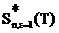=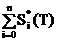, when n = 0,1,2, derived from (15) solving the problem sketched in Section 2 (see  for further details). Note that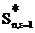is the (n+1)-th partial sum of the series expansion (15) evaluated in e=1, n=0,1,…. Recall that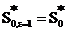is the Barone-Adesi Whaley approximation of the free boundary. Table 1 shows that roughly for n = 0,1 going from the n-th order approximation to (n+1)-th order approximation of the solution one correct significant digit is added to the free boundary approximation obtained. This effect is particularly evident when b>0 in fact in this case the Barone-Adesi, Whaley approximation of the free boundary is poor and has no correct significant digits. Note that positive values of b correspond to values of the continuous dividend yield d = r-b smaller than the risk free interest rate r. Recall that in the case of American call options when b>0 and r-b>0 the integral equation satisfied by the free boundary deduced in  becomes singular as  0+.

When r=0.08, s = 0.2, b = -0.04,  and  t = T  Table 2 shows the asset price S, the European call option price CE obtained evaluating the Black Scholes formula in S, t, and the approximations of the American call option price in S, t  obtained using the trinomial method CT (i.e. CT is the ``true value" of the option price used as benchmark), the Barone-Adesi, Whaley formula CBW and the n-th order approximation CA,n,e = 1, n = 1,2, derived from (14), (15), (8) using the method presented in . Furthermore we show the relative errors that follows:

reBW(S,T) = |CBW(S,T)-CT(S,T)|/CT(S,T),                (16)

and

reCn,e = 1(S,T) = |CA,n,e = 1(S,T)-CT(S,T)|/CT(S,T),      n = 1,2.      (17)

Table 1: Approximations of the free boundary of an American call option with intermediate maturity T and strike price E = 100.

 r=0.08,  s=0.2,  b=-0.04 T S*(T)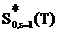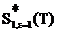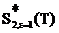3 129.065 12.88 129.036 128.951 5 131.064 130.701 131.154 130.973 10 132.687 32.207 132.862 132.705 r=0.08,  s=0.2,   =0.00 T S*(T)3 149.676 150.206 149.017 149.617 5 155.051 156.97 154.473 154.823 10 160.505 164.206 160.463 160.129 r=0.08,  s=0.2,  b=0.04 T S*(T)3 241.191 255.316 236.006 242.394 5 251.915 273.499 246.402 252.762 10 265.960 304.17 260.422 265.212

Table 2: Approximations of the price of an American call option with intermediate maturity T, strike price E = 100 and negative cost of carrying.

 r=0.08  s=0.2,  b=-0.04 maturity T = 3 S CE(S,T) CT(S,T) CBW(S,T) CA,1,e = 1(S,T) CA,2,e = 1(S,T) reCBW(S,T) reC1,e = 1(S,T) reC2,e = 1(S,T) 70 0.8211 0.9587 1.0861 0.9258 0.9617 1.33·10-1 3.38·10-2 3.58·10-3 80 1.9285 2.3414 2.5258 2.3068 2.3334 7.87·10-2 1.47·10-2 3.41·10-3 90 3.7480 4.7585 4.9706 4.7193 4.7336 4.45·10-2 8.24·10-3 5.23·10-3 100 6.3571 8.4921 8.6777 8.4453 8.4504 2.18·10-2 5.49·10-3 4.91·10-3 110 9.7526 13.792 13.896 13.746 13.748 7.52·10-3 3.34·10-3 3.18·10-3 120 13.872 20.886 20.906 20.878 20.882 1.04·10-3 2.88·10-4 1.11·10-4 maturity T = 5 S CE(S,T) CT(S,T) CBW(S,T) CA,1,e = 1(S,T) CA,2,e = 1(S,T) reCBW(S,T) reC1,e = 1(S,T) reC2,e = 1(S,T) 70 1.1410 1.5349 1.7261 1.5612 1.4912 1.24·10-1 1.71·10-2 2.84·10-2 80 2.2026 3.1467 3.3887 3.1927 3.1021 7.68·10-2 1.46·10-2 1.14·10-2 90 3.7323 5.6921 5.9446 5.7503 5.6506 4.43·10-2 1.02·10-2 7.28·10-3 100 5.7491 9.4043 9.6129 9.4629 9.3695 2.21·10-2 6.22·10-3 3.70·10-3 110 8.2416 14.523 14.640 14.570 14.498 8.05·10-3 3.27·10-3 1.71·10-3 120 11.178 21.302 21.319 21.330 21.288 8.13·10-4 1.32·10-3 6.15·10-4 maturity T = 10 S CE(S,T) CT(S,T) CBW(S,T) CA,1,e = 1(S,T) CA,2,e = 1(S,T) reCBW(S,T) reC1,e = 1(S,T) reC2,e = 1(S,T) 70 1.0638 2.1845 2.3679 2.2715 2.1878 8.39·10-2 3.98·10-2 1.47·10-3 80 1.7276 3.9387 4.1329 4.0428 3.9483 4.93·10-2 2.64·10-2 2.43·10-3 90 2.5807 6.5377 6.7081 6.6482 6.5526 2.60·10-2 1.68·10-2 2.26·10-3 100 3.6212 10.201 10.312 10.302 10.216 1.08·10-2 9.94·10-3 1.51·10-3 110 4.8422 15.166 15.198 15.246 15.179 2.11·10-2 5.29·10-3 8.71·10-4 120 6.2334 21.690 21.666 21.746 21.705 1.11·10-3 2.59·10-3 7.02·10-4

Note that Tables 1 and 2 show that increasing the approximation order of the solution of the American call option pricing problem obtained from the expansions in powers of  e (14), (15) studied in  and mentioned in Section 2 (that is increasing n)  it is possible to improve substantially the results obtained with the Barone-Adesi, Whaley formula (i.e. the result obtained when n = 0).

Figure 1 shows the ``true" free boundary, the Barone-Adesi, Whaley free boundary, the first and the second order approximations of the free boundary obtained with the expansions (14), (15) developed in  and sketched in Section 2 as a function of t, 0<t<T, when E = 100, T = 10, r = 0.08, s = 0.2, d = 0.04 (b=r-d). This figure  shows that the first order approximation of the free boundary improves significantly the zero-th order approximation of the free boundary (i.e. the Barone-Adesi, Whaley free boundary) and that the second order approximation of the free boundary refines the result obtained with the first order approximation.  Movie 1  shows the ``true" free boundary, the Barone-Adesi, Whaley free boundary, the first and the second order approximations of the free boundary obtained with the expansions developed in  and sketched in Section 2  when E = 100, T = 10, r = 0.08,  s = 0.2 as a function of the time to maturity t,  0<t<T,  for d=r-b, b=bi=-0.06+i0.006, i=0,1,2…20.  That is for i=1,2,...,20 the i-th frame of Movie 1 shows the American call option free boundary as a function of time to maturity t,  0<t<T, T=10, obtained using the integral equation  deduced in  called  “true free boundary”,  the  Barone-Adesi, Whaley formula, and the first and second order approximations resulting from the truncation of  the power series expansions deduced in  when b= bi.

Movie 1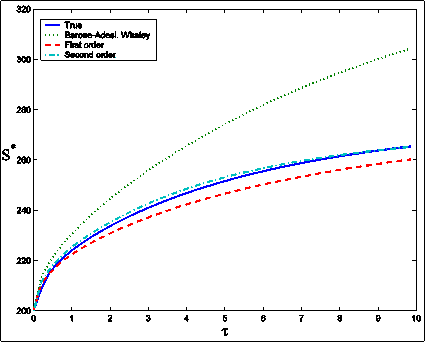Figure 1: American call option free boundary S* as a function of the time to maturity t, 0< t<T, when E = 100, T = 10, r = 0.08, s = 0.2, b = 0.04: ``true" free boundary (solid line), Barone-Adesi, Whaley free boundary (dotted line), first order approximation of the free boundary (dashed line), second order approximation of the free boundary (dash-dotted line).

Figures 2 (a), 2(b) show the ``true" and the approximated prices of the American call option corresponding to the free boundaries shown in Figure 1 as a function of the time to maturity t, 0 £ t £ T, T=10, when the asset price S takes the values S = 90 (Figure 2 (a)) and S = 110 (Figure 2 (b)). The approximated option prices are obtained using the zero-th, first and second order approximations obtained truncating the series expansions deduced in  and sketched in Section 2. Figures 1 and 2 suggest that the second order corrections of the option price and of the free boundary are necessary to have satisfactory numerical solutions of the American call option pricing problem when we consider at time t=0 or  at time t close to zero options with intermediate maturity times (i.e. 3 £ T £ 10).  Movie 2 illustrates the relation between the three approximations mentioned above of the American call option price. In particular  Movie 2  shows the American call option prices  as a function of the time to maturity for a fixed value of the asset price obtained using the trinomial tree method (called “true price”), the  Barone-Adesi, Whaley formula, and the first and second order approximations resulting from the truncation of the power series expansions (14), (15) studied in . The parameter  values of the American call option pricing problem considered in Movie 2 are those of Figure 2(a), 2(b), that is dividend yield d=0.04 (b=r-d=0.04), interest rate r=0.08, volatility s=0.2, asset price S=90, time to maturity t between 0 and 10 years, strike price E=100.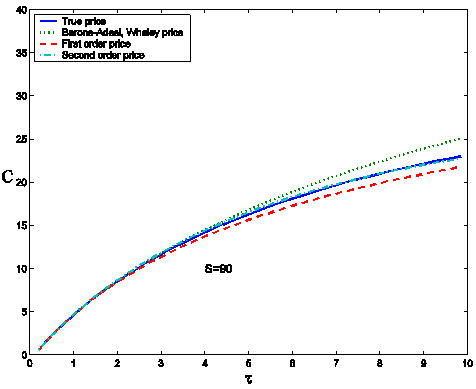(a)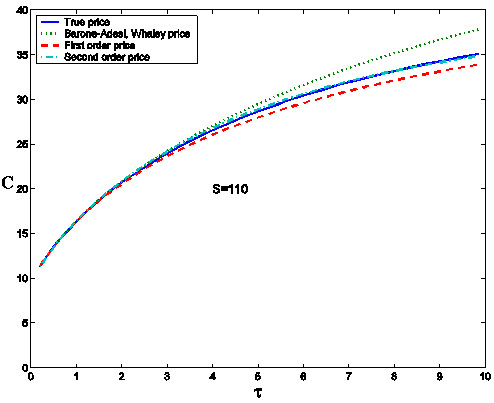Figure 2(b)

Figure 2: American call option price C as a function of the time to maturity t for two values of the asset price S = 90 (a), S = 110 (b), when E = 100, T = 10, r = 0.08, s= 0.2, b = 0.04. ``True" price CT (solid line), Barone-Adesi, Whaley price CBW (dotted line), first order approximation CA,1,e = 1 of the price (dashed line), second order approximation CA,2,e = 1 of the price (dash-dotted line).

4. An interactive application and an app

An interactive application to solve the American option pricing problem with the Barone-Adesi, Whaley formula and with the first and second order approximations obtained truncating the series expansions (14), (15) developed in  and sketched in Section 2 is provided. The interactive application takes as input data the volatility s, the risk free interest rate r, the dividend yield d, the strike price E, the  asset price S, the time to maturity t and the type (call or put) of the American option considered.

The output of the interactive application is the price and the free boundary of the American option pricing problem corresponding to the values S and t assigned  computed using the Barone-Adesi, Whaley formula and the first and second order approximations obtained truncating the series expansions (14), (15).

The  app is a simple translation of the interactive application in the Android environment. The appis freely downloadable  and runs on devices based on the Android software system.  For example it can be used on  Android smart phones and tablets.

5. The importance of the accuracy of the free boundary approximation: a numerical experiment

We present a numerical experiment that shows the relevance of having an accurate approximation  of the free boundary for the holder of an American option. Let us recall that the values of the parameters that define the experiment are: E = 100, T = 10, r = 0.08, s = 0.2, d = 0.04,  b=r-d=0.04. We consider the American call option pricing problem corresponding to these parameter values  whose free boundary as a function of the time to maturity is shown in Figure 1.

Let us recall that we work in the Black Scholes framework and that in this framework we have a market where there are no arbitrage opportunities,  no transaction costs and the asset price dynamics is given (1).

We consider four investors holding the American call option specified above. We suppose that the first investor knows the “true” value of the free boundary and that the last three investors know only an approximation of it. That is the second, third and fourth investor knows, respectively, the zero-th, first and second order approximations of the free boundary obtained truncating the series expansions (14), (15) with the method sketched in Section 2. These four investors sell the option when the underlying asset price reaches the free boundary known to them and capitalize the revenue of the sale (i.e. the option price) from the time of the sale up to time T at the risk free interest rate.  Note that these four investors adopt the decision of selling the option when the underlying asset price reaches the free boundary, that is they adopt the optimal decision suggested by the mathematical analysis of the American option pricing problem.

More specifically in the experiment  we simulate 10000 trajectories with a time step Dt=0.001(years) of the price, St, t=ti=iDt, i=1,2,…,10000, starting from an initial asset value S0 at t=0 that is smaller than the “true” free boundary and than the approximated free boundaries known to the investors considered. Each investor checks at t= ti , i=1,2,…, 10000, whether the simulated asset price is greater than the free boundary known to him. When this is true for the first time at  t=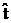(time of the sale) the investor exercises the option and invests the revenue of the sale, that is the money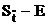in the market at the risk free interest rate r (r=0.08) from the time of the saleup to time t=T=10 (years).  That is, the investor at time t=T gets an amount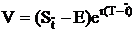. When the option is not exercised the investor at time t=T gets an amount V equal to the value of the option at time t=T.  Let us denote with Vtrue, VBW, V1, V2 the value of V obtained by the four investors at  maturity time T. In Figure 3 we show the averages over 10000 trajectories of the asset price dynamic equation mentioned above of the quantities  (Vtrue-VBW )/Vtrue ,  (Vtrue-V1 )/Vtrue  and (Vtrue-V2 )/Vtrue, versus the moneyness  S0/E. We denote these averages as follows: rBW=E((Vtrue-VBW )/Vtrue ),  r1=E((Vtrue-V1 )/Vtrue ) and r2=E((Vtrue-V2 )/Vtrue), where E(∙) denotes the expected value of ∙. Roughly speaking, the quantities rBW,  r1 and r2   are the average percentage of loss of the American call option investor that knows the approximations of the free boundary obtained using, respectively, the Barone-Adesi, Whaley formula, the first and second order approximations of the free boundary sketched in Section 2.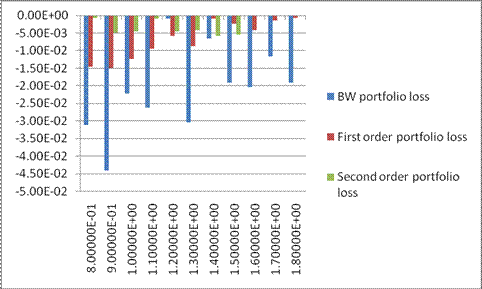Figure 3:  Percentage of loss rBW=E((Vtrue-VBW )/Vtrue ), r1=E((Vtrue-V1)/Vtrue ) and r2=E((Vtrue-V2 )/Vtrue) of the three holders that know only an approximation of the free boundary for different values of the moneyness  S0/E.

## 6. References

   Barone-Adesi, G.,  Whaley, R.E. (1987). Efficient analytical approximation of American option values. The Journal of Finance, Vol. 42(2), 301-320.

  Ju, N., Zhong, R. (1999).  An approximate formula for pricing American options. The Journal of Derivatives,  Vol. 7(2), 31-40.

  Little, T., Pant, V., Hou, C. (2000). A new integral representation of the early exercise boundary for American put options. Journal of Computational Finance, Vol. 3, 73-96.

  Fatone, L.,  Mariani, F.,  Recchioni,  M.C.,  Zirilli, F.: The Barone-Adesi Whaley  formula to price American options revisited,   in preparation.

 Boyle, P. (1986). Option Valuation using three-jump process. International Options Journal, Vol. 3, 7-12.

  Secovic, D. (2001). Analysis of the free boundary for the pricing of an American call option. European Journal of Applied Mathematics, Vol. 12(1),

25 - 37.

Entry n.  2608